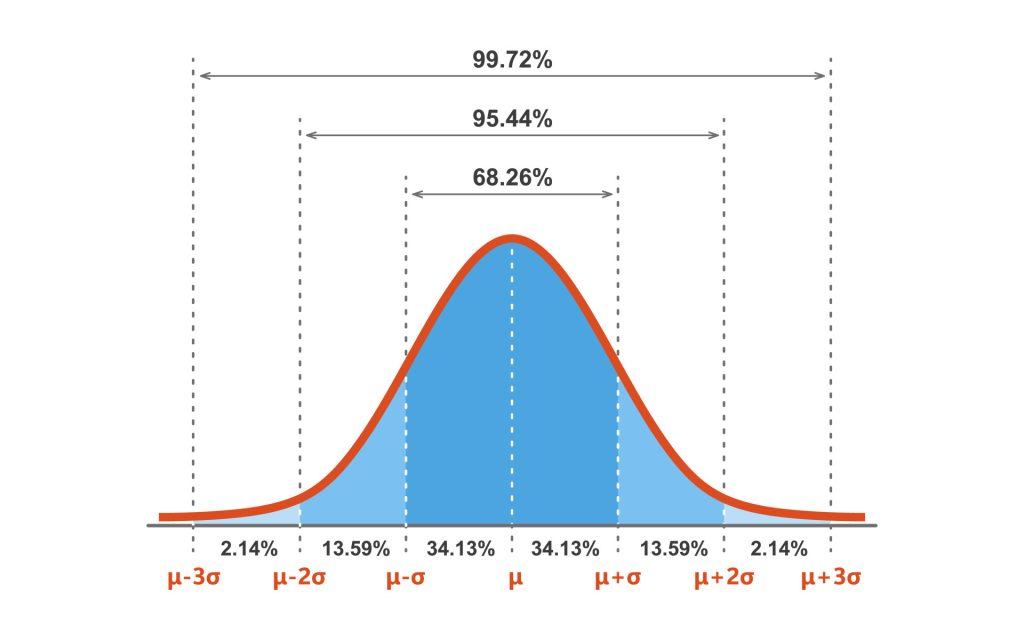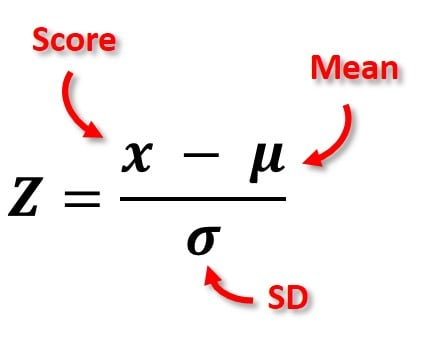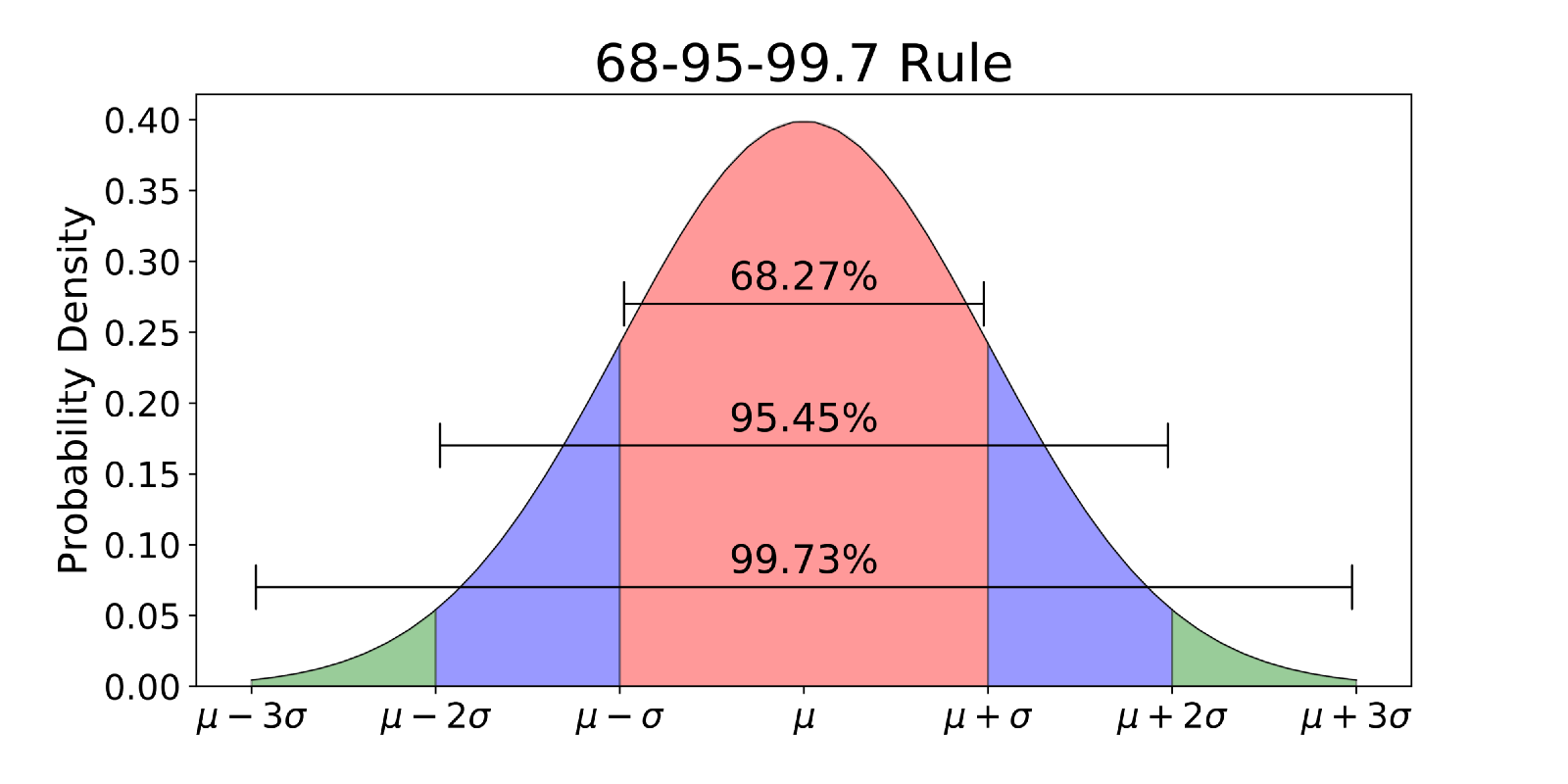# Z-Score: Definition, Formula, Calculation & Interpretation

A z-score describes the position of a raw score in terms of its distance from the mean when measured in standard deviation units. The z-score is positive if the value lies above the mean and negative if it lies below the mean.

It is also known as a standard score because it allows the comparison of scores on different kinds of variables by standardizing the distribution. A standard normal distribution (SND) is a normally shaped distribution with a mean of 0 and a standard deviation (SD) of 1 (see Fig. 1).Figure 1. A standard normal distribution (SND).

## Why are z-scores important?

It is useful to standardize the values (raw scores) of a normal distribution by converting them into z-scores because:

(a) it allows researchers to calculate the probability of a score occurring within a standard normal distribution;

(b) and enables us to compare two scores that are from different samples (which may have different means and standard deviations).

## How to calculate

The formula for calculating a z-score is z = (x-μ)/σ, where x is the raw score, μ is the population mean, and σ is the population standard deviation.

As the formula shows, the z-score is simply the raw score minus the population mean, divided by the population standard deviation.Figure 2. Z-score formula in a population.

When the population mean and the population standard deviation are unknown, the standard score may be calculated using the sample mean (x̄) and sample standard deviation (s) as estimates of the population values.

## Interpretation

The value of the z-score tells you how many standard deviations you are away from the mean. If a z-score is equal to 0, it is on the mean.

A positive z-score indicates the raw score is higher than the mean average.

For example, if a z-score is equal to +1, it is 1 standard deviation above the mean.

A negative z-score reveals the raw score is below the mean average. For example, if a z-score is equal to -2, it is 2 standard deviations below the mean.

Another way to interpret z-scores is by creating a standard normal distribution (also known as the z-score distribution or probability distribution).

## Standard Normal Distribution (SND)

1. The SND (i.e., z-distribution) is always the same shape as the raw score distribution. For example, if the distribution of raw scores is normally distributed, so is the distribution of z-scores.
2. The mean of any SND always = 0.
3. The standard deviation of any SND always = 1. Therefore, one standard deviation of the raw score (whatever raw value this is) converts into 1 z-score unit.

The SND allows researchers to calculate the probability of randomly obtaining a score from the distribution (i.e., sample). For example, there is a 68% probability of randomly selecting a score between -1 and +1 standard deviations from the mean (see Fig. 4).Figure 4. The proportion of a standard normal distribution (SND) in percentages.

The probability of randomly selecting a score between -1.96 and +1.96 standard deviations from the mean is 95% (see Fig. 4). If there is less than a 5% chance of a raw score being selected randomly, then this is a statistically significant result.

## Calculating a raw score

Sometimes we know a z-score and want to find the corresponding raw score. The formula for calculating a z-score in a sample into a raw score is given below:

X = (z)(SD) + mean

As the formula shows, the z-score and standard deviation are multiplied together, and this figure is added to the mean.

Check your answer makes sense: If we have a negative z-score, the corresponding raw score should be less than the mean, and a positive z-score must correspond to a raw score higher than the mean.

## Calculating a z-score using excel

To calculate the z-score of a specific value, x, first, you must calculate the mean of the sample by using the AVERAGE formula.

For example, if the range of scores in your sample begins at cell A1 and ends at cell A20, the formula =AVERAGE(A1:A20) returns the average of those numbers.

Next, you must calculate the standard deviation of the sample by using the STDEV.S formula. For example, if the range of scores in your sample begins at cell A1 and ends at cell A20, the formula = STDEV.S (A1:A20) returns the standard deviation of those numbers.

Now to calculate the z-score, type the following formula in an empty cell: = (x – mean) / [standard deviation].

To make things easier, instead of writing the mean and SD values in the formula, you could use the cell values corresponding to these values. For example, = (A12 – B1) / [C1].

Then, to calculate the probability for a SMALLER z-score, which is the probability of observing a value less than x (the area under the curve to the LEFT of x), type the following into a blank cell: = NORMSDIST( and input the z-score you calculated).

To find the probability of LARGER z-score, which is the probability of observing a value greater than x (the area under the curve to the RIGHT of x), type: =1 – NORMSDIST (and input the z-score you calculated).Olivia Guy-Evans

BSc (Hons), Psychology, MSc, Psychology of Education

Associate Editor for Simply Psychology

Olivia Guy-Evans is a writer and associate editor for Simply Psychology. She has previously worked in healthcare and educational sectors.Saul Mcleod, PhD

Educator, Researcher

BSc (Hons) Psychology, MRes, PhD, University of Manchester

Saul Mcleod, Ph.D., is a qualified psychology teacher with over 18 years experience of working in further and higher education.